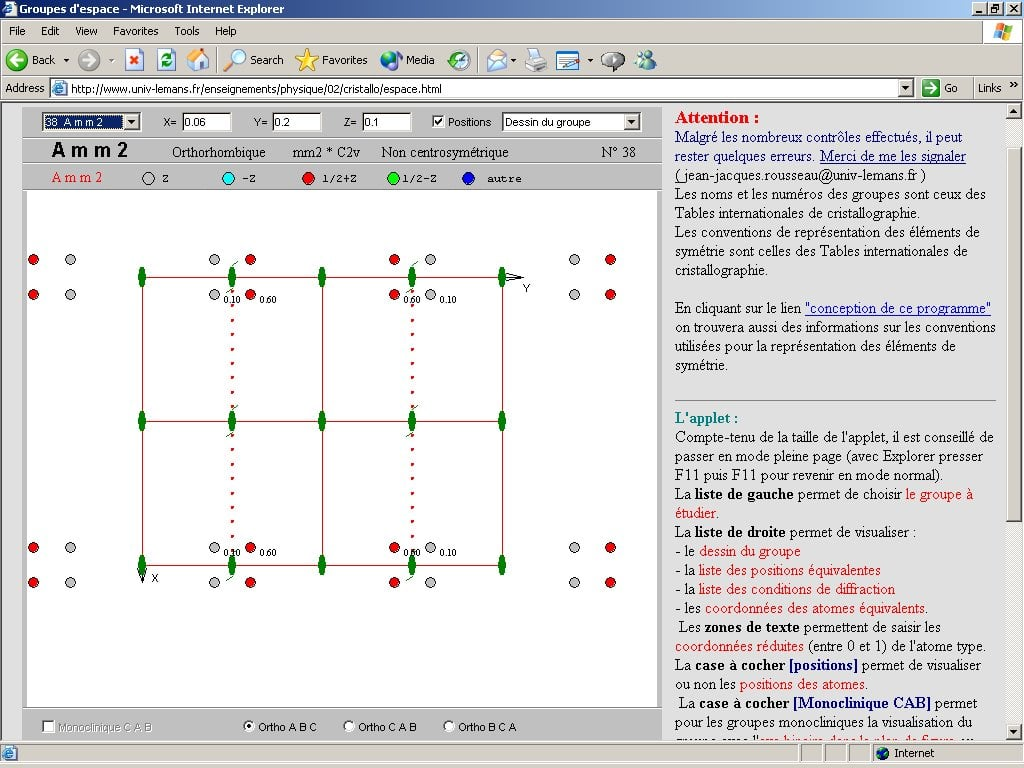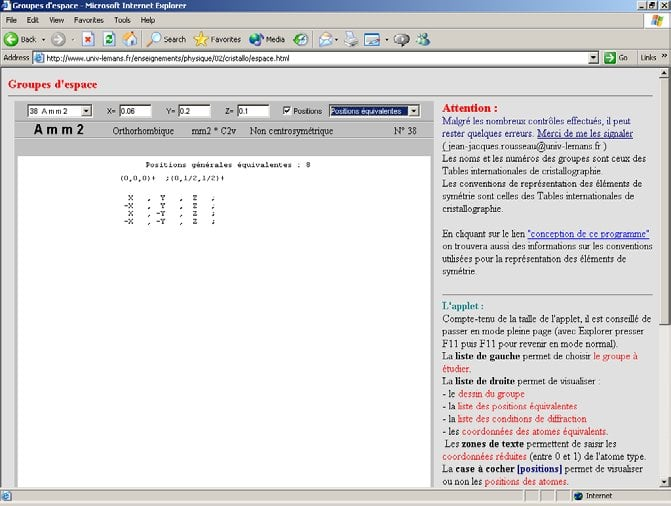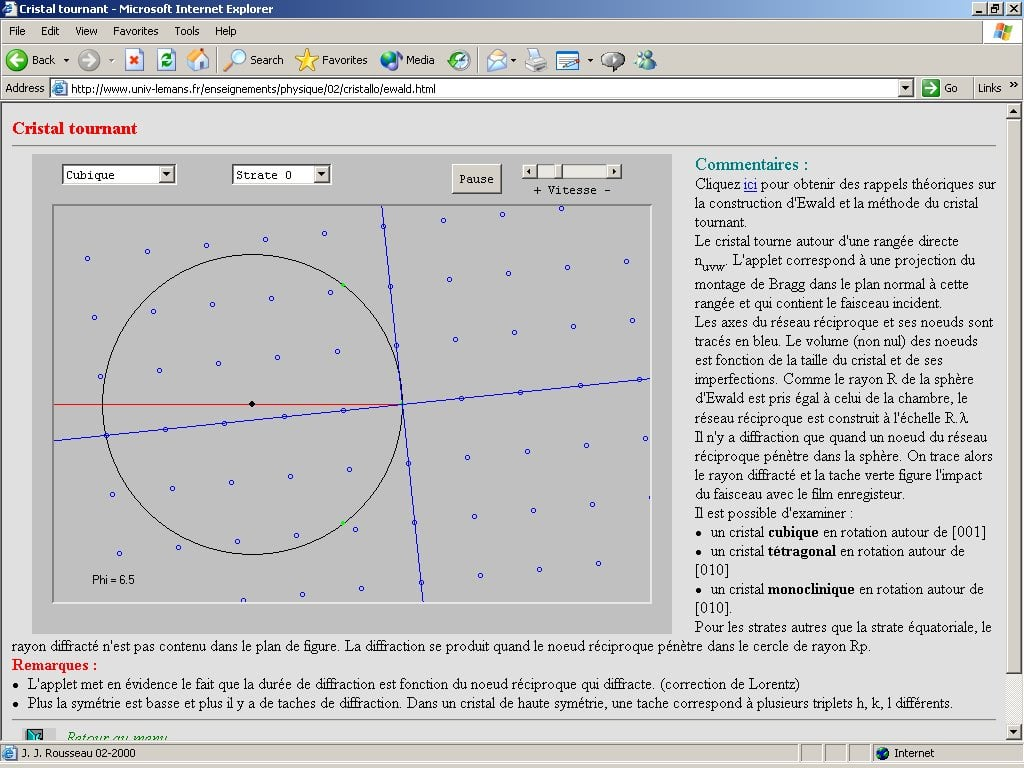# Use of JAVA applets for teaching

Jean-Jacques Rousseau,

Université du Maine, Le Mans, France.
E-mail:
jjrouss@univ-lemans.fr – WWW: http://www.univ-lemans.fr/enseignements/physique/02/

## Why use Java for teaching?

• It is a portable language which generates code for a virtual machine: if the browser installed on the computer (PC, Mac...) is equipped with a Java virtual machine, it is possible to download and exploit the applets. These applets use, in a transparent way for the programmer, the graphic objects (buttons, elevators/sliders, etc...) of the operating system.
• It is a reliable and protected language : an applet cannot write on the user hard disc.
• It is a language equipped with simple but sufficient graphic capacities.
• It is a language which allows the realization of graphic animations
• The mathematical library respects the IEEE 754 standards and allows scientific computation.
• It is very simple to incorporate applets in a HTML formatted page.
• The Java syntax is very close to that of C. Java is very strict with the types of data and it is a strongly object oriented language. For simple applications, one can use it like a traditional language.
• Finally, I made the choice, for reasons of maximum compatibility, to use only the Java versions 1.0 and 1.1. To use the later versions which are admittedly equipped with higher graphic capacities, most browsers will ask for a "plug-in"which is usually easy to find and install.

## The applet "space groups"

This applet (approximately 3500 lines of code) which gives the chart of the 230 space groups is available at the address:

http://www.univ-lemans.fr/enseignements/physique/02/cristallo/espace.html

## Applet design

For each selected space group, the program carries out 4 operations:

• Layout of the symmetry elements.
• Determination of the equivalent positions in literal form.
• Calculation and layout of the equivalent positions.
• Determination of the diffraction conditions.

## Layout of the symmetry elements

This layout is carried out starting from a table where the elementary generators of each space group are coded. The application of some simple rules on lattice translation and on the nature of the elements of symmetry of the space group makes it possible to reduce this table.

For all the centrosymetric groups, the origin is taken on a centre of inversion allowing to simplify later calculations of the equivalent positions.

For some cubic groups, elements of symmetry were voluntarily omitted for clarity.

As an example, here is how the three representations of the Amm2 space group are coded:

G="MV000090 MV000000 CV002500 200000 210025";

H="MV000090 MH0000 BH1025 02001090 1200209025";

K="MH0000 MV000000 AV002500 02000000 12002500";

CV000090 => C = mirror c, V = vertical, 00 and 25 = coordinates (in 1/100) of the origin from the mirror, 00 = angle (in degree) of the mirror with Ox.

1200209025 => 12 = horizontal axis 21, 00 = passes by the origin, 20 = to draw in 2nd position, 90 = angle in degrees of the axis with Ox, 25 = dimension of the axis into 1/100.

210025 => vertical axis 21 which passes by the point of coordinates 00/100 and 25/100.Fig. 1: Screen image of the space groups applet showing the drawing of symmetry elements of Amm2.

## Determination of the equivalent positions in literal form

In the calculation of the equivalent positions, one separates the effect of the operations of symmetry of the specific point group from the influences of the translations. After decoding of the Herman-Mauguin symbol of the space group, one determines by application of the point symmetry operators how the coordinates of the node [1, 1, 1] change. The 3x3 matrices thus obtained for each equivalent position are preserved in a table (matrix) S. In the same way a table T (vector) contains the sum of the intrinsic translations related to the symmetry elements (screw axes and glide planes) and of the translations related to the position of the symmetry elements in the reference system used.

The initial triplet X (vector of components x, y, z) is transformed into a triplet X' according to the matrix relation X' = S.X + T

The analysis of the components of tables S and T allows the drawing up of the table of the equivalent general positions in literal form.

The translations related to the lattice mode are then treated globally for displaying the equivalent positions. Finally, for each position thus determined, one adds for the realization of the diagram the integer lattice translations (1, 0, 0) (-1, 0, 0) (0, 1, 0) (0, -1, 0) (1, 1, 0) (-1, 1, 0) (1, -1, 0) (-1, -1, 0).

During the displaying of the list of the literal values, one specifies at the beginning of this list the lattice translations which must be possibly added. The generators used are not always the same ones as those of the International Tables for Crystallography, or are used in a different order. This is why the order of the displayed list can differ from that of the Tables.

## Calculation and layout of the equivalent positions

In the calculation of the equivalent positions in numerical form, the user must enter the reduced coordinates of the initial atom. The program calculates the list of the numerical coordinates of the equivalent atoms starting from the list of the literal coordinates and carries out the layout on the screen.

If the atom is in a special position, the program generates two (4, 8, ...) atoms having the same coordinates. A procedure makes it possible to eliminate the redundant atoms and to display only one atom: this allows the study of the special positions. All calculations are carried out in reduced coordinates. For the layouts, the conversion between these crystallographic coordinates and the screen coordinates takes account of the system under study.

For the trigonal, hexagonal and cubic space groups, it is often interesting to modify the default values of the coordinates in order to obtain a more readable projection.Fig. 2: Screen image of the space groups applet showing the output of equivalent positions for Amm2.

Note: An atom is in special position if it is placed on a non-screwed rotation axis or in a non-glide mirror. In the International Tables, the special positions are listed and marked by their Wyckoff symbol (number of equivalent positions followed by an allotted letter in a conventional way).

## Determination of the diffraction conditions

The determination of the systematic extinctions for general positions is made starting from the analysis of the contents of table T. The program displays the list of the conditions on the indices of the reticular planes which lead to diffraction. The first line of display corresponds to the conditions suitable for the lattice mode. The next lines correspond to the conditions related to the elements of symmetry of translation of the group. The extinctions which are induced by atoms in special positions (on one or more elements of symmetry without translation) are not calculated.

## An animation: the Ewald construction

For making my students appreciate all the subtleties of the Ewald construction, I wrote the small program available at the address:

http://www.univ-lemans.fr/enseignements/physique/02/cristallo/ewald.htmlFig. 3: Screen image of the Ewald construction applet.

It is an animation in which I rotate a plane of the reciprocal lattice in the plane of the figure. When a node penetrates the Ewald sphere, the diffracted beam is traced and a spot is drawn on the film.

To obtain fluid animations, without flickering, I highly recommend the method of the double-buffer. See for example (but it is in French):

http://www.univ-lemans.fr/enseignements/physique/02/java/mnujava.html

## How does an animation function?

It is necessary to start by defining a "thread" with the instruction:

Then it is necessary to implement the methods start(), stop() and run().

public void start ()

{ if (runner == null) {

runner.start( ); }}

public void stop()

{ if (runner ! = null) {

runner.stop( );

runner = null;}}

public void run( )

{ while (true)

{ try {

repaint( );